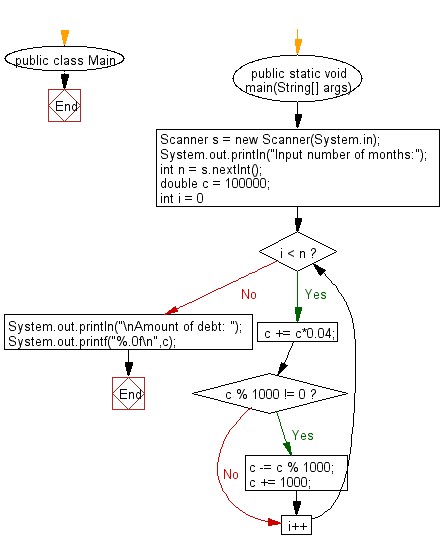# Java Exercises: Compute the amount of the debt in n months

## Java Basic: Exercise-215 with Solution

Write a Java program to compute the amount of the debt in n months. The borrowing amount is \$100,000 and the loan adds 4% interest of the debt and rounds it to the nearest 1,000 above month by month.

Input:

An integer n (0 ≤ n ≤ 100).

Sample Solution:

Java Code:

``````import java.util.Scanner;
public class Main {
public static void main(String[] args) {
Scanner s = new Scanner(System.in);
System.out.println("Input number of months:");
int n = s.nextInt();
double c = 100000;
for(int i = 0; i < n; i++) {
c += c*0.04;
if(c % 1000 != 0) {
c -= c % 1000;
c += 1000;
}
}
System.out.println("\nAmount of debt: ");
System.out.printf("%.0f\n",c);
}
}
```
```

Sample Output:

```Input number of months:
6

Amount of debt:
129000
```

Flowchart:Java Code Editor:

What is the difficulty level of this exercise?

﻿

## Java: Tips of the Day

Parsing dates:

```import java.io.*;
import java.util.*;
import java.text.*;

String s = "2001/09/23 14:39";

SimpleDateFormat formatter = new SimpleDateFormat ("yyyy/MM/dd H:mm");
Date d = formatter.parse(s, new ParsePosition(0));
```